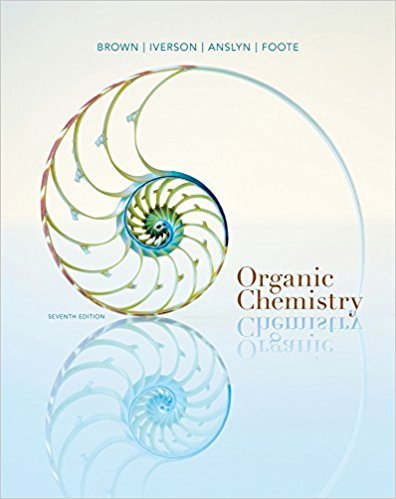×
Get Full Access to Organic Chemistry - 7 Edition - Chapter 4 - Problem 4.18
Get Full Access to Organic Chemistry - 7 Edition - Chapter 4 - Problem 4.18

×

# If the DG0 for a reaction is 24.5 kcal/mol at 298 K, what is the Keq for this reactionISBN: 9781133952848 483

## Solution for problem 4.18 Chapter 4

Organic Chemistry | 7th Edition

• Textbook Solutions
• 2901 Step-by-step solutions solved by professors and subject experts
• Get 24/7 help from StudySoup virtual teaching assistantsOrganic Chemistry | 7th Edition

4 5 1 275 Reviews
10
2
Problem 4.18

If the DG0 for a reaction is 24.5 kcal/mol at 298 K, what is the Keq for this reaction? Whatis the change in entropy of this reaction if DH0 5 23.2 kcal/mol?

Step-by-Step Solution:
Step 1 of 3

ICE Tables Friday, January 26, 2018 3:50 PM K pK cRT) . n N = change of moles of GAS K p Equilibrium constant for pressure K c Equilibrium constant for concentration R = 0.08201 Lxatm/molxK T = Temperature in Kelvin Q - the ratio pf products to reactants (to the power of their stoichiometric coefficients) of concentrations or activity at any give time If Q < eq ---> not enough products If Q = eq ---> reaction is at equilibrium If Q > eq ---> Too many products ICE = Initial Change Equilibrium Reaction: N 2 O 2--> 2NO In this problem, we start with 2 mol of N and O 2 2 N2 O2 2NO Initial 2 2 0 Change -x -x +2x Equilibrium 2-x 2-x 2x Kc= [NO] / [N2][NO2] 2 2 4 = 2x /(2-x) Le Chatelier's Principle ICE Tables Friday, January 26, 2018 3:50 PM K pK cRT) . n N = change of moles of GAS K p Equilibrium constant for pressure K c Equilibrium constant for concentration R = 0.08201 Lxatm/molxK T = Temperature in Kelvin Q - the ratio pf products to reactants (to the power of their stoichiometric coefficients) of concentrations or activity at any give time If Q < eq ---> not enough products If Q = eq ---> reaction is at equilibrium If Q > eq ---> Too many products ICE = Initial Change Equilibrium Reaction: N 2 O 2--> 2NO In this problem, we start with 2 mol of N and O

Step 2 of 3

Step 3 of 3

##### ISBN: 9781133952848

This textbook survival guide was created for the textbook: Organic Chemistry, edition: 7. This full solution covers the following key subjects: . This expansive textbook survival guide covers 24 chapters, and 1131 solutions. Since the solution to 4.18 from 4 chapter was answered, more than 263 students have viewed the full step-by-step answer. Organic Chemistry was written by and is associated to the ISBN: 9781133952848. The answer to “If the DG0 for a reaction is 24.5 kcal/mol at 298 K, what is the Keq for this reaction? Whatis the change in entropy of this reaction if DH0 5 23.2 kcal/mol?” is broken down into a number of easy to follow steps, and 32 words. The full step-by-step solution to problem: 4.18 from chapter: 4 was answered by , our top Chemistry solution expert on 03/16/18, 05:02PM.

## Discover and learn what students are asking

Calculus: Early Transcendental Functions : Functions of Several Variables
?In Exercises 15 - 22, find all first partial derivatives. $$f(x, y)=\frac{x y}{x+y}$$

Calculus: Early Transcendental Functions : Slope Fields and Eulers Method
?In Exercises 1-8, verify the solution of the differential equation. Solution D

Calculus: Early Transcendental Functions : Area and Arc Length in Polar Coordinates
?In Exercises 5-16, find the area of the region. One petal of $$r=2 \cos 3 \theta$$

Statistics: Informed Decisions Using Data : The Normal Probability Distribution
?Find the value of z0.20.

Statistics: Informed Decisions Using Data : Tests for Independence and the Homogeneity of Proportions
?The table in the next column contains observed values and expected values in parentheses for two categorical variables, X and Y, where variable X has

Unlock Textbook Solution